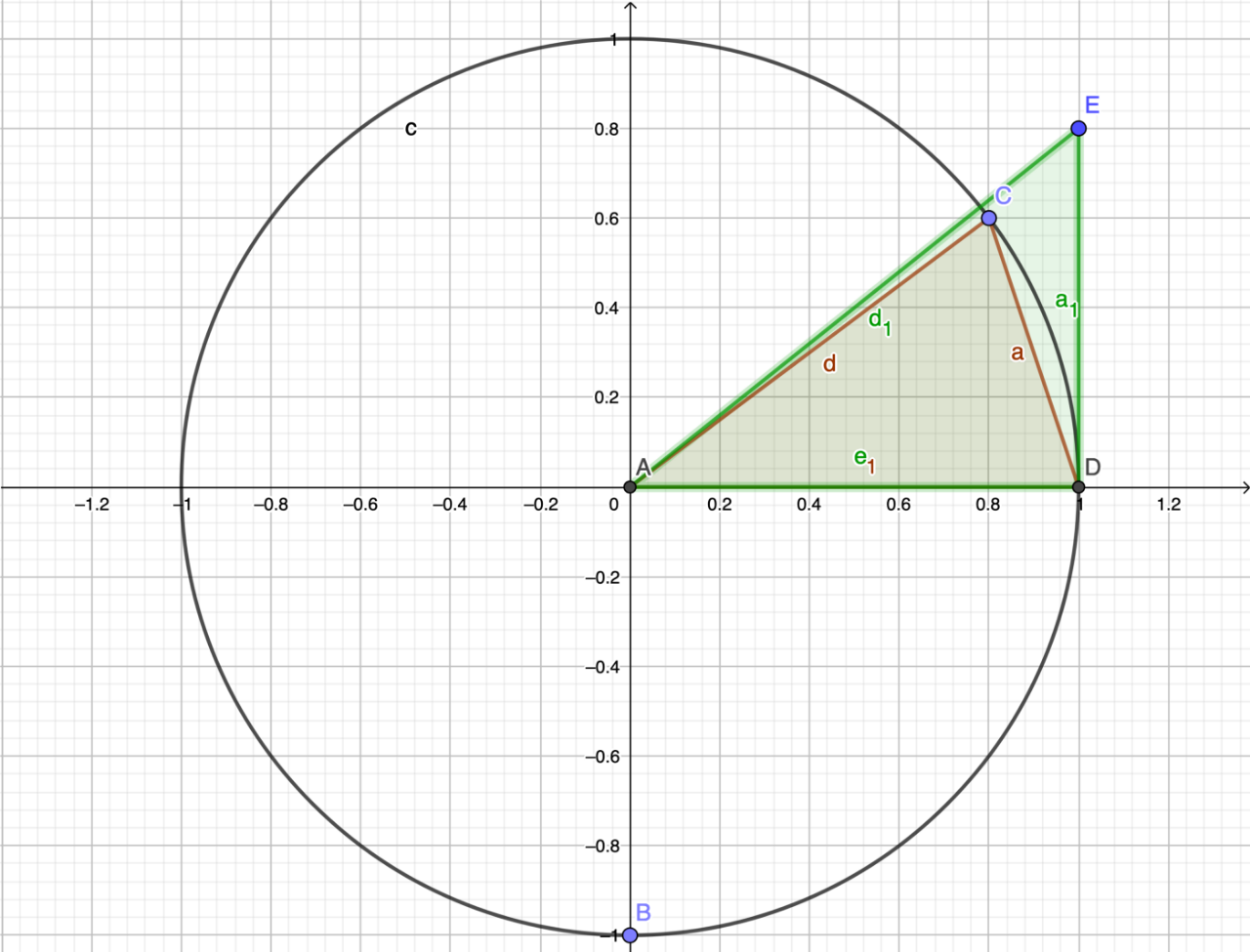# A proof of the Squeeze Theorem (and why I like it)

An intuitive explanation of an awesome theorem.

While I was studying calculus, I came across this statement:

!!\lim _{\theta \rightarrow 0} \frac{\sin \theta}{\theta}=1!!

This amazed my because of the fact that sin of 0 is 0, so with substitution of theta in the fraction next to the limit, it would end up as 0/0 or undefined. Now, I had already studied limits a bit and knew about l’hopital’s rule. So, I proceeded to take the derivative of the top and bottom of the fraction with respect to theta and checked out what I got. This got me to the expected answer of 1/1 = 1 because the derivative of sin(x) is cos(x) and cos(0) is 1. Now this was all fine and dandy, but as I did more research into the limit listed above, I found a theorem called The Squeeze Theorem which I thought was a much more fun way to solve this same problem.

## The Proof

To begin, take a look at this picture of a few triangles drawn using different points around a unit circle. If you don’t already know, a unit circle is one with a radius of 1. The point A is at (0,0), point C is at some point on the edge of the circle, point D is at (1,0), and point E is at some point (1, x).Using this visual, let us compare the areas of two triangles and a sector.

!!△ACD \le ⌔ACD \le △AED!!

We can see that this is true by just looking at the graph. Next, I will define the values of the areas. Note that theta will refer to the angle made on the unit circle with each of the shapes (the bottom left angle of the triangle or sector).

!!\begin{array}{c}{\triangle ACD=\frac{1}{2} b h=\frac{1}{2} \cdot 1 \cdot \sin \theta=\frac{\sin \theta}{2}} \\ {⌔ACD=\frac{1}{2} r^{2} \theta=\frac{1}{2} \cdot 1^{2} \cdot \theta=\frac{\theta}{2}} \\ {\triangle AED=\frac{1}{2} b h=\frac{1}{2} \cdot 1 \cdot \tan \theta=\frac{\tan \theta}{2}}\end{array}!!

Just FYI, the hight of triangle △AED is tan(x) because tan(x) is opposite over adjacent and adjacent is 1, so it is really just the side opposite the angle, which is the hight. (That confused me at first too 😂)

Now that we have this, we can re-compare (is that a word?) the areas using actual values.

!!\frac{\sin \theta}{2} \leq \frac{\theta}{2} \leq \frac{\tan \theta}{2}!!

Next, I am going to perform a set of operation to all of the elements in the inequality. This is legal as it preserves the truthfulness of the inequality like applying the same operation to each side of an equals sign preserves the equality.

First, I will multiply each element by 2.

!!\sin \theta \leq \theta \leq \tan \theta!!

Second, I will divide by sin(𝜃).

!!1 \leq \frac{\theta}{\sin \theta} \leq \frac{1}{\cos \theta}!!

Finally, I will take the reciprocal of each.

!!1 \geq \frac{\sin \theta}{\theta} \geq \cos \theta!!

These reciprocals will get us to the final result that we want. All we have to do now is take the limit as listed at the top and analyze the results.

!!\begin{array}{c}{\lim _{\theta \rightarrow 0}\left[1 \geq \frac{\sin \theta}{\theta} \geq \cos \theta\right]} \\ {\lim _{\theta \rightarrow 0}\left[1 \geq \frac{\sin \theta}{\theta} \geq 1\right]} \\ {1 \geq \lim _{\theta \rightarrow 0} \frac{\sin \theta}{\theta} \geq 1}\end{array}!!

As you can most likely see (I highlighted it), by substitution of the limit value into each element of the inequality, we get 1 is greater than or equal to the limit of sin theta over theta as theta approaches 0 which is greater than or equal to 1.

Because we know this:

!!\begin{array}{c}{\text {if}} \\ {1 \geq x \geq 1} \\ {\text {then,}} \\{x=1}\end{array}!!

Now we can say that the limit of sin of theta over theta as theta approaches 0 has to be 1 because of what I just stated above. Kinda cool, right?